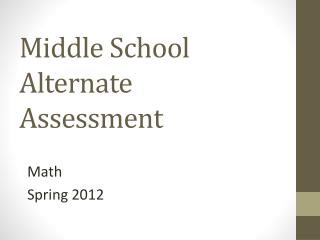Download PresentationMiddle School Alternate Assessment

# Middle School Alternate Assessment

Download Presentation## Middle School Alternate Assessment

- - - - - - - - - - - - - - - - - - - - - - - - - - - E N D - - - - - - - - - - - - - - - - - - - - - - - - - - -
##### Presentation Transcript

1. Middle School Alternate Assessment Math Spring 2012

3. Expression Match

6. Use a Geoboard and start with a square that is one unit on each side. Have students use rubber bands to create squares that are 2, 3, 4, and even 5 units on each side. Then, find the area of each square. Do the same with triangles and other quadrilaterals.

7. Example—6th Grade Dot Plot X X X X XXX X XXXXX 14 15 16 17 18 19 20 Ages of the students in our class

8. Example—6th Grade Ages of the students in our class 14 15 16 17 18 19 20 How old are most students in your class?How do you know?

11. Use the inchworms manipulative. They come in 1 inch, 2 inch, 3 inch, and 4 inch lengths. Have a picture of anything. (You can place it on 1 inch grid paper if needed.) Use the inchworms to measure in inches. Use a scale of 1 inch = 2 feet (or other appropriate scale). Find the actual lengths of the object in the picture.

12. 1 cm = 2 feet

13. Divide into rectangles to determine area. A= 21 cm2 B= 5cm2 C= 18cm2 Total Area= 45 cm2

15. Activities

16. 7th Grade • Define volume. “Volume measures space. It is the amount of cubic units that takes up a space.” • Demonstrate by pouring water into a container. • Point out household items that are measured in volume (milk carton, bottle of cola, box of sugar cubes, sandbox, etc.) • Have students look through magazines and cut out pictures of items and discuss how to talk about each item’s volume. • Stress “cubed”. Note: Volume is NOT weight.

17. Volume 5cm3

18. Activities • Fill a box with cubes (wooden, plastic, or sugar cubes). • Count the number of cubes it takes to fill up this rectangular prism with cubes. • Discuss the volume as “cubed”. • Students fill up different boxes with cubes and compare the volumes. APPS: --Geometry: Volume of Solids

19. Activities • Relate to Base 10 Set. • Show a 1000s cube. Have students stack hundreds squares on top of each other to make the cube. How many does it take? How many unit cubes does it take to fill up the larger cube? APPS: --Base Ten Number Blocks (\$0.99)

20. Activities • Use rectangular baking pans and wet or dry ingredients. • Determine how many cups (of water, sugar, flour, etc.) it takes to fill up that pan. • A cup is another unit of volume.

21. Face • Part of a shape that is flat.(Or curved) • For example, A cube has 6 of these.

22. Surface Area: the measure of all the sides How many pairs of identical faces does cube have? Calculate the area of each face. Add up the areas to determine total surface area. A cube has 6 identical faces. Each face is a square. Each face of the cube has sides which are 9 inches long. The area of one face is 9 in x 9in = 81 square inches There are 6 faces: 81 x 6 = 486 square inches.

23. IXL Surface Area, IXL.com

24. Activities • Surface area is the area of all of the sides added together. • Take a box and break it apart to show all 6 sides. (This is called a “net”.) • Measure or label the length of each side. • Find the area of each individual rectangle then add them up to find total surface area. • Stress “Covers” and “Square units”. How much do we need to cover the whole box? • You could have students wrap a box to find out how much wrapping paper covers the box.

25. Activities

26. Activities • Draw the net of a cube on grid paper. • Students count the blocks to find the area of each part. • Add up all the parts to get the total surface area. • Do this on cardstock and cut out then fold and tape to make the cube.

27. Activities Activity 7

28. Using the Accucut

30. These triangles are similar.

31. These ovals are similar.

32. Real-life Similar Shapes Sunshine Skyway Bridge Tampa, Florida

33. Real-life Similar Shapes

35. Complete the Function Table. spider spiders 24 48 72

36. Answer dog 2 4 6

37. Rule: ___________ Teacher provides input values and a rule, while student determines output values.

38. Rule: ____________

39. Spin a Rule

40. Spin a Rule

41. Complete the Function Table. At Larry’s Lemonade Stand, 1 cup of lemonade costs \$ 0.50. Complete the function table to show the cost of lemonade. Can you determine the rule? ______________